# P Block - Group 17 Questions and Answers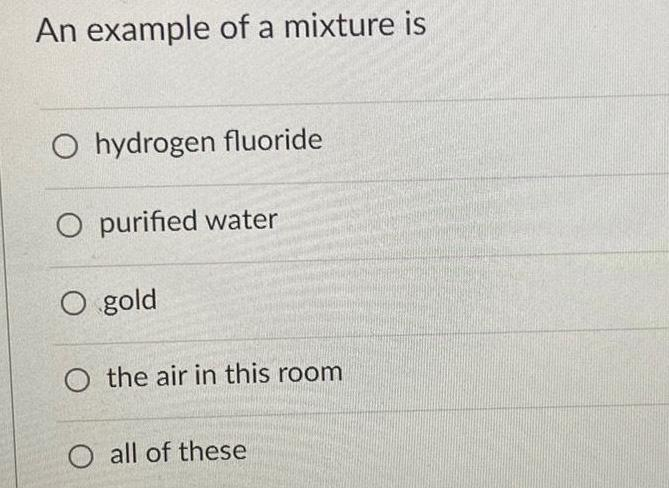Inorganic Chemistry
P Block - Group 17
An example of a mixture is hydrogen fluoride purified water gold the air in this room all of these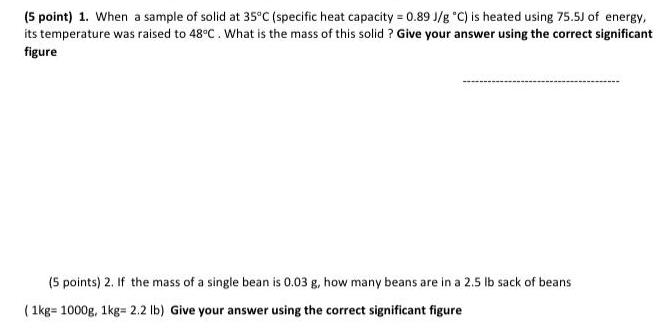Inorganic Chemistry
P Block - Group 17
When a sample of solid at 35°C (specific heat capacity = 0.89 J/g °C) is heated using 75.5J of energy, its temperature was raised to 48°C. What is the mass of this solid? Give your answer using the correct significant figure If the mass of a single bean is 0.03 g, how many beans are in a 2.5 lb sack of beans (1kg=1000g, 1kg= 2.2 lb) Give your answer using the correct significant figure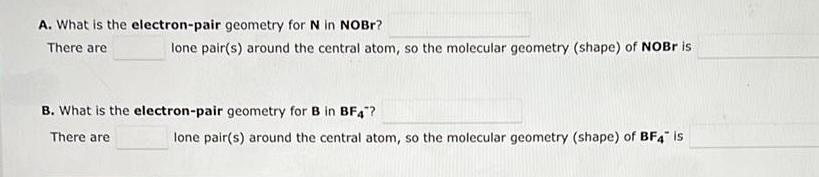Inorganic Chemistry
P Block - Group 17
A. What is the electron-pair geometry for N in NOBr? There are ___ lone pair(s) around the central atom, so the molecular geometry (shape) of NOBr is ___ B. What is the electron-pair geometry for B in BF4-? There are ___ lone pair(s) around the central atom, so the molecular geometry (shape) of BF4- is ___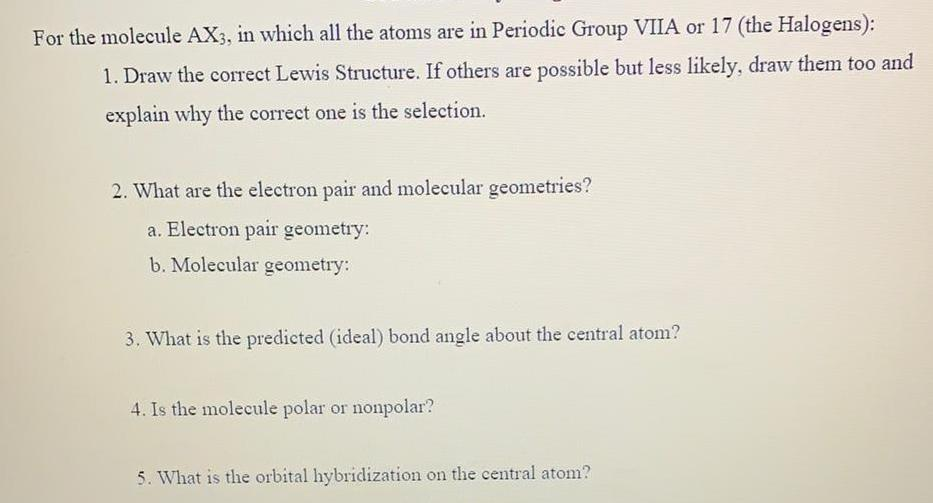Inorganic Chemistry
P Block - Group 17
For the molecule AX3, in which all the atoms are in Periodic Group VIIA or 17 (the Halogens): 1. Draw the correct Lewis Structure. If others are possible but less likely, draw them too and explain why the correct one is the selection. 2. What are the electron pair and molecular geometries? a. Electron pair geometry: b. Molecular geometry: 3. What is the predicted (ideal) bond angle about the central atom? 4. Is the molecule polar or nonpolar? 5. What is the orbital hybridization on the central atom?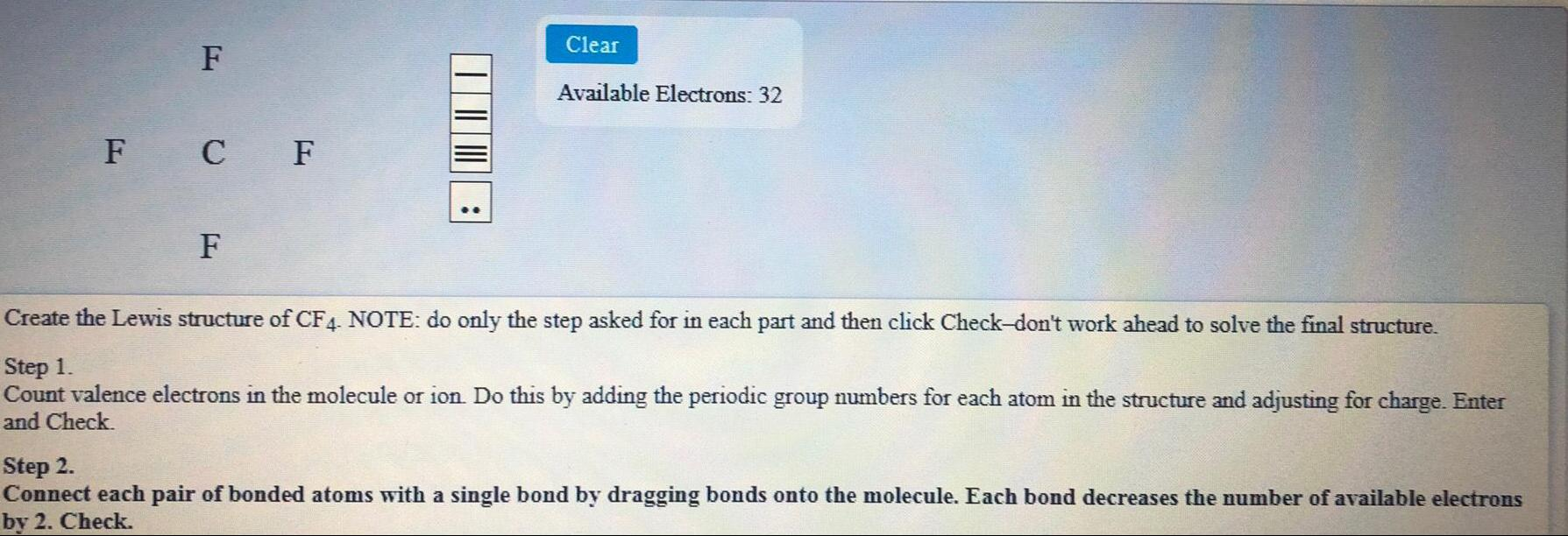Inorganic Chemistry
P Block - Group 17
Create the Lewis structure of CF44 NOTE: do only the step asked for in each part and then click Check-don't work ahead to solve the final structure. Step 1. Count valence electrons in the molecule or ion. Do this by adding the periodic group numbers for each atom in the structure and adjusting for charge. Enter and Check. Step 2. Connect each pair of bonded atoms with a single bond by dragging bonds onto the molecule. Each bond decreases the number of available electrons by 2. Check.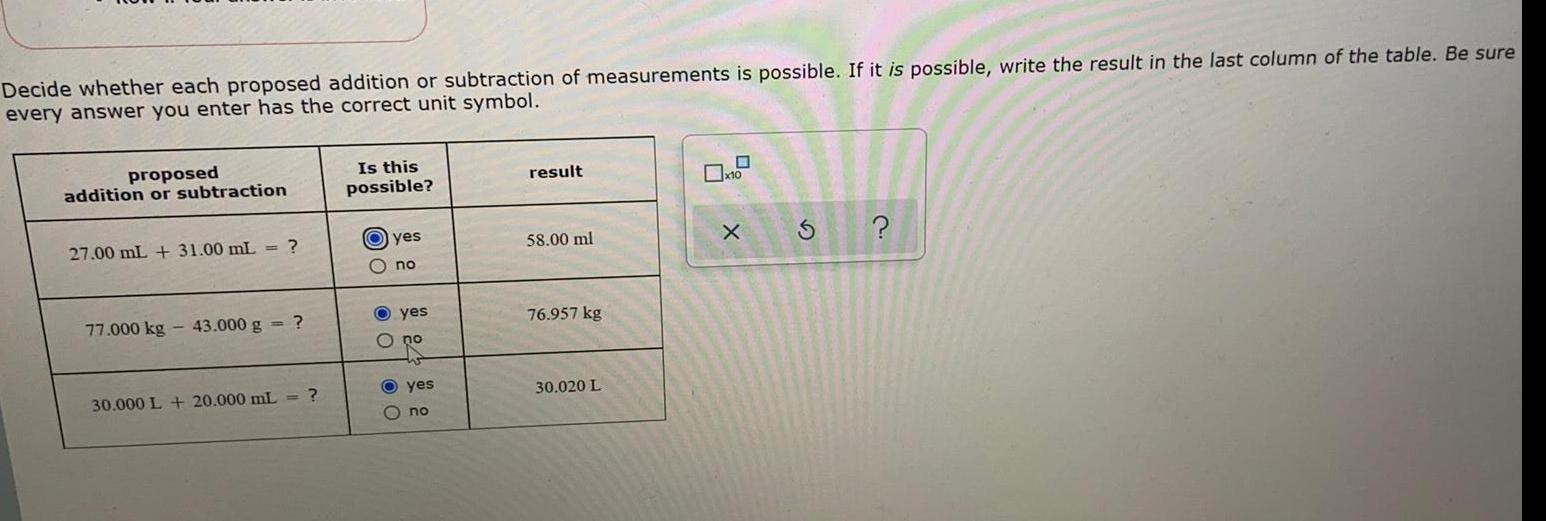Inorganic Chemistry
P Block - Group 17
Decide whether each proposed addition or subtraction of measurements is possible. If it is possible, write the result in the last column of the table. Be sure every answer you enter has the correct unit symbol. proposed addition or subtraction 27.00 mL + 31.00 mL = ? 77.000 kg - 43.000 g = ? 30.000 L + 20.000 mL = ?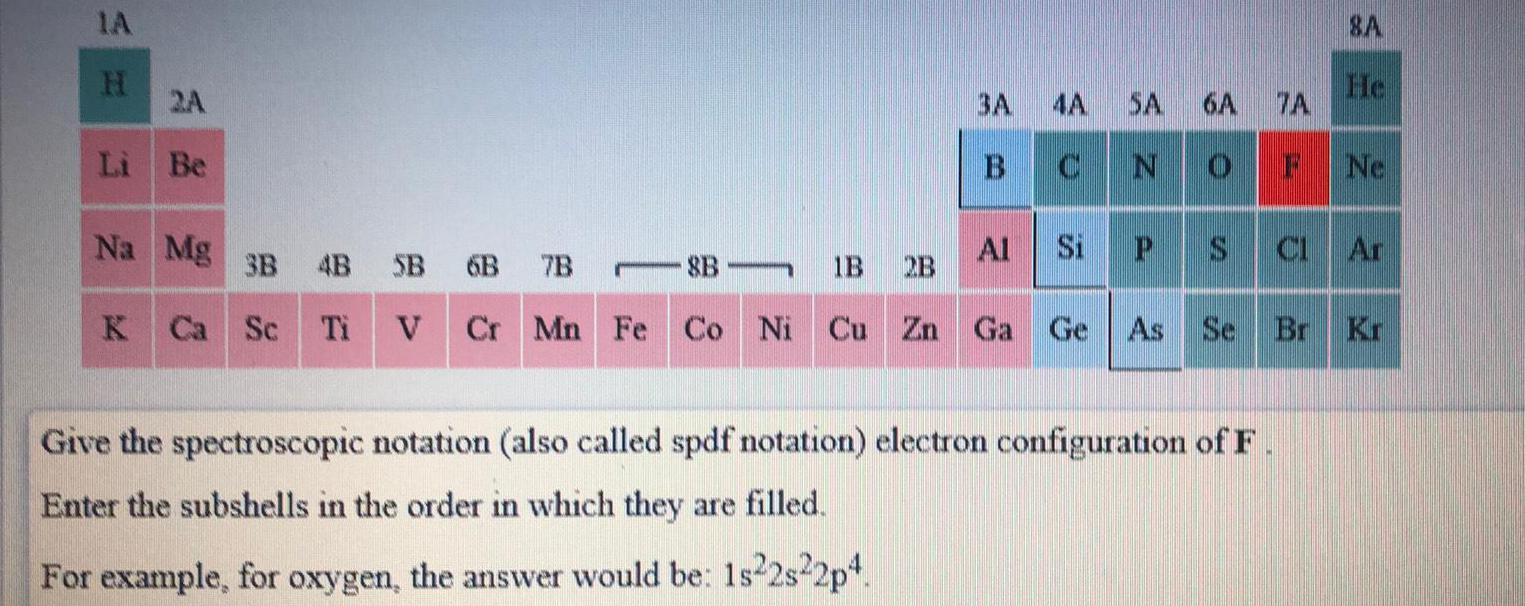Inorganic Chemistry
P Block - Group 17
Give the spectroscopic notation (also called spdf notation) electron configuration of F Enter the subshells in the order in which they are filled. For example, for oxygen, the answer would be: 1s22s22p4.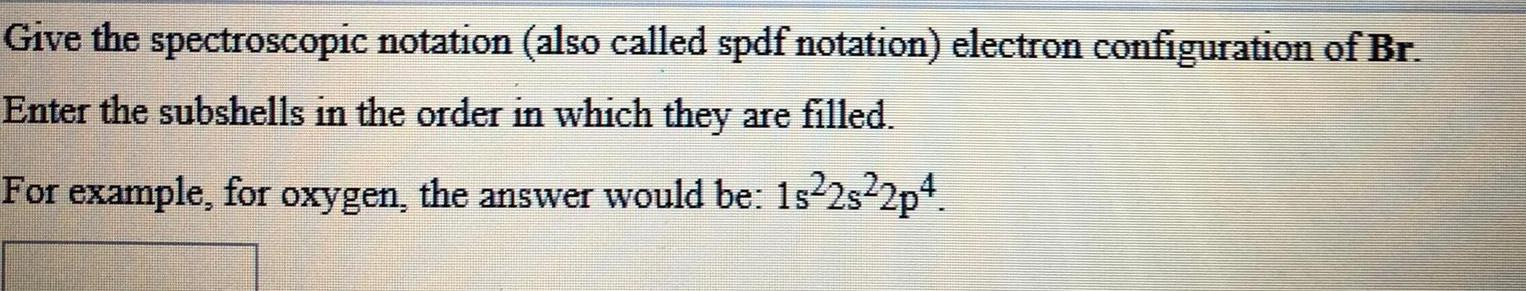Inorganic Chemistry
P Block - Group 17
Give the spectroscopic notation (also called spdf notation) electron configuration of Br. Enter the subshells in the order in which they are filled. For example, for oxygen, the answer would be: 1s²2s²2p4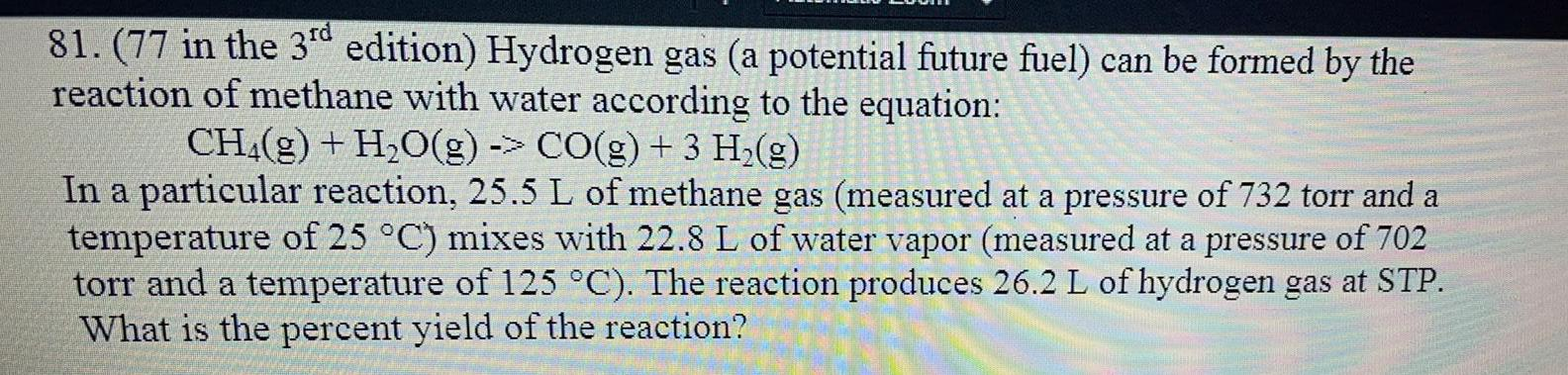Inorganic Chemistry
P Block - Group 17
81. (77 in the 3rd edition) Hydrogen gas (a potential future fuel) can be formed by the reaction of methane with water according to the equation: CH4(g) + H₂O(g) -> CO(g) + 3 H₂(g) In a particular reaction, 25.5 L of methane gas (measured at a pressure of 732 torr and a temperature of 25 °C) mixes with 22.8 L of water vapor (measured at a pressure of 702 torr and a temperature of 125 °C). The reaction produces 26.2 L of hydrogen gas at STP. What is the percent yield of the reaction?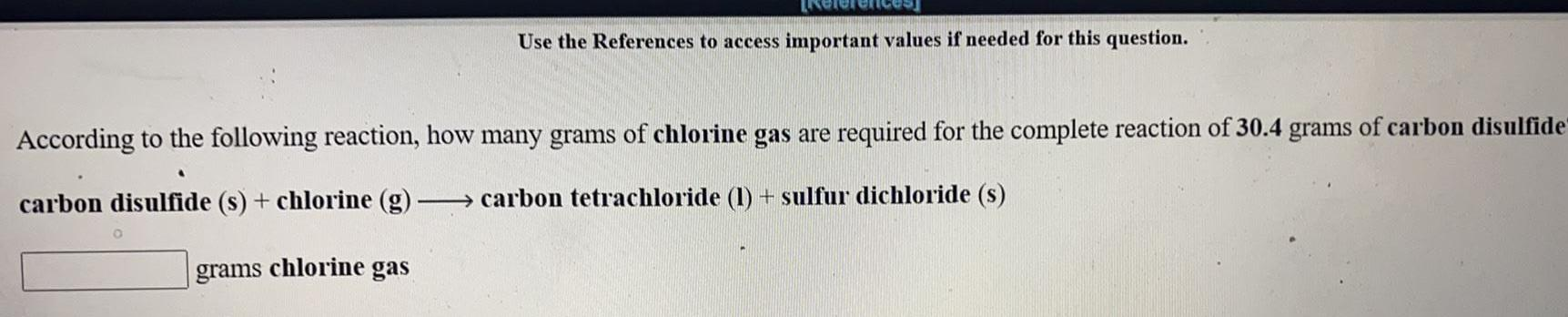Inorganic Chemistry
P Block - Group 17
According to the following reaction, how many grams of chlorine gas are required for the complete reaction of 30.4 grams of carbon disulfide carbon disulfide (s) + chlorine (g) -> carbon tetrachloride (1) + sulfur dichloride (s)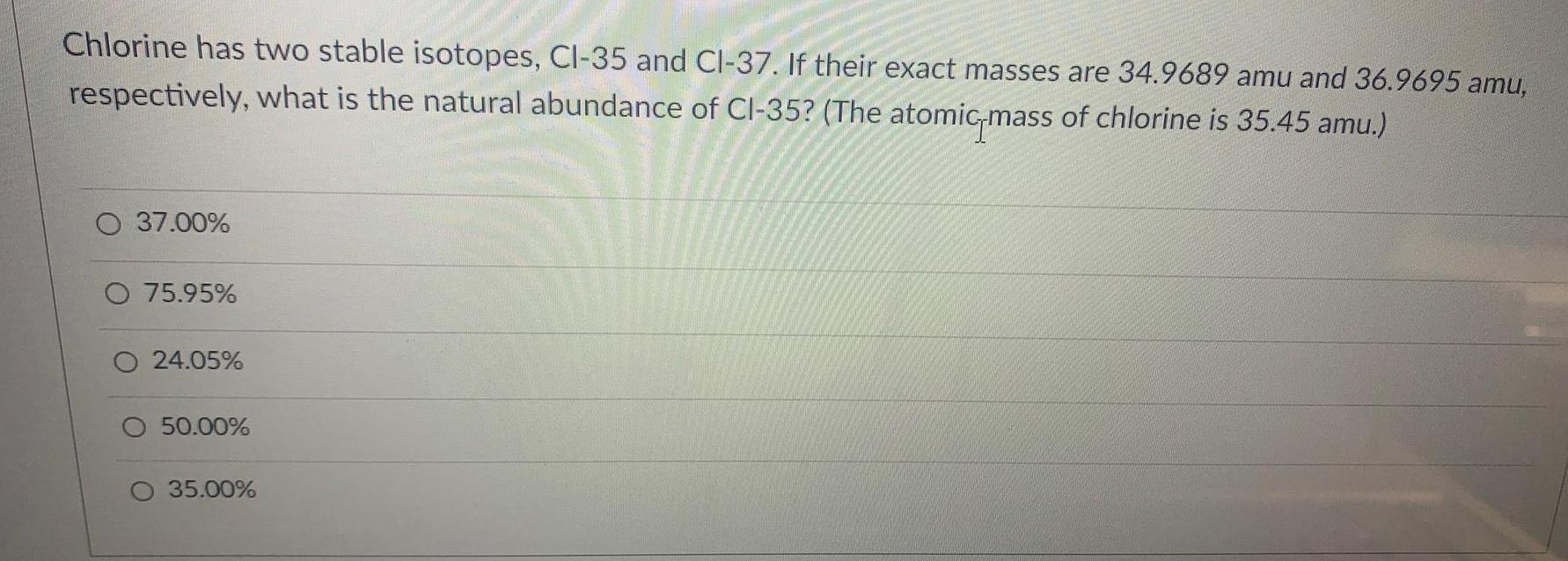Inorganic Chemistry
P Block - Group 17
Chlorine has two stable isotopes, Cl-35 and Cl-37. If their exact masses are 34.9689 amu and 36.9695 amu, respectively, what is the natural abundance of Cl-35? (The atomic-mass of chlorine is 35.45 amu.) 37.00% 75.95% 24.05% 50.00% 35.00%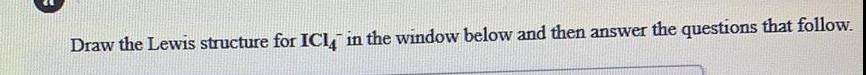Inorganic Chemistry
P Block - Group 17
Draw the Lewis structure for ICI4-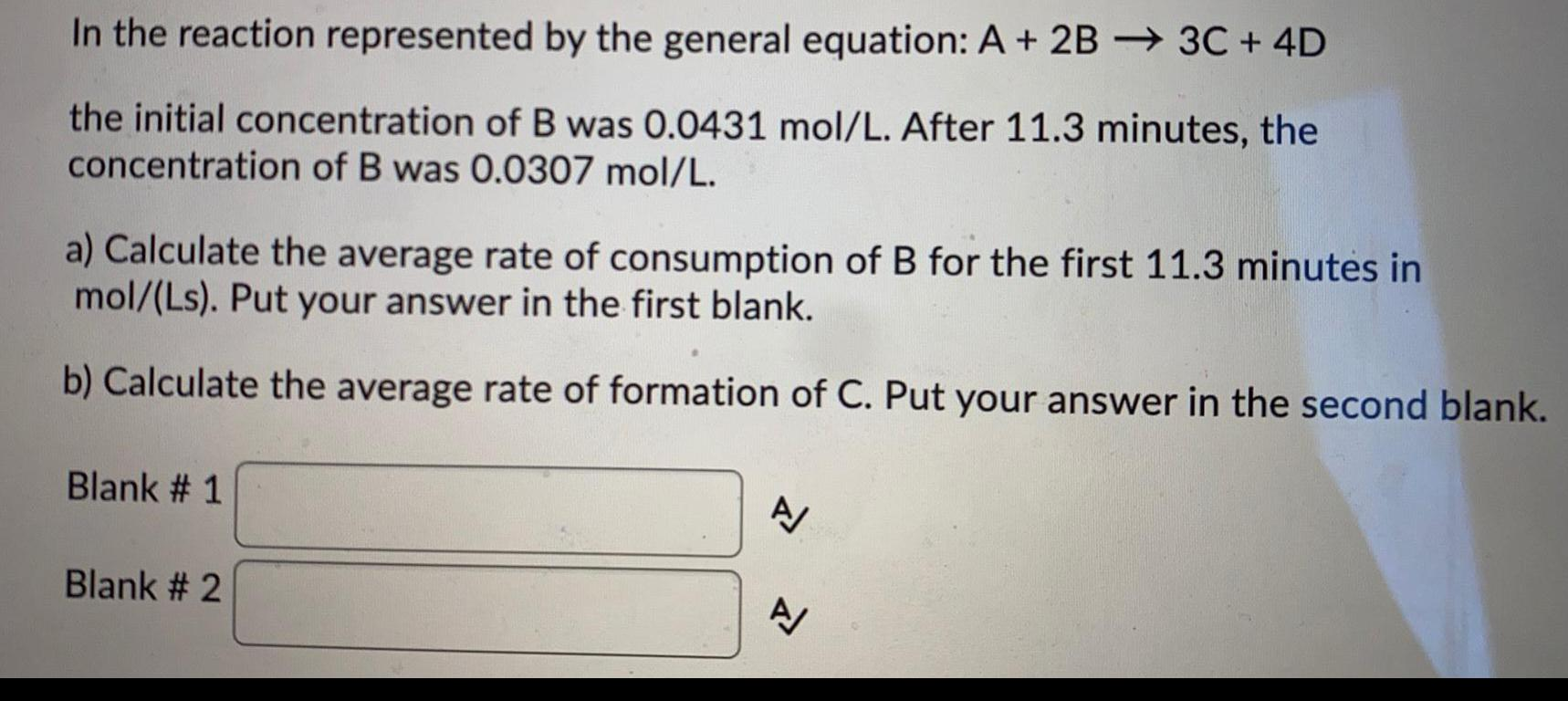Inorganic Chemistry
P Block - Group 17
In the reaction represented by the general equation: A + 2B 3C + 4D the initial concentration of B was 0.0431 mol/L. After 11.3 minutes, the concentration of B was 0.0307 mol/L. a) Calculate the average rate of consumption of B for the first 11.3 minutes in mol/(Ls). Put your answer in the first blank. b) Calculate the average rate of formation of C. Put your answer in the second blank.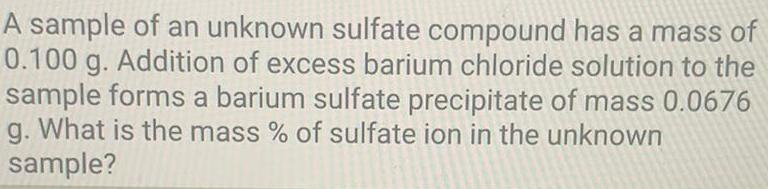Inorganic Chemistry
P Block - Group 17
A sample of an unknown sulfate compound has a mass of 0.100 g. Addition of excess barium chloride solution to the sample forms a barium sulfate precipitate of mass 0.0676 g. What is the mass % of sulfate ion in the unknown sample?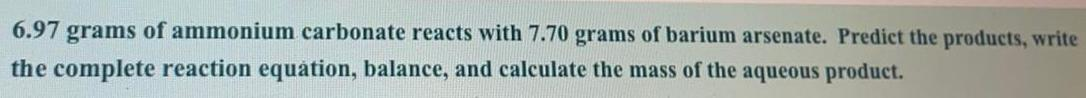Inorganic Chemistry
P Block - Group 17
6.97 grams of ammonium carbonate reacts with 7.70 grams of barium arsenate. Predict the products, write the complete reaction equation, balance, and calculate the mass of the aqueous product.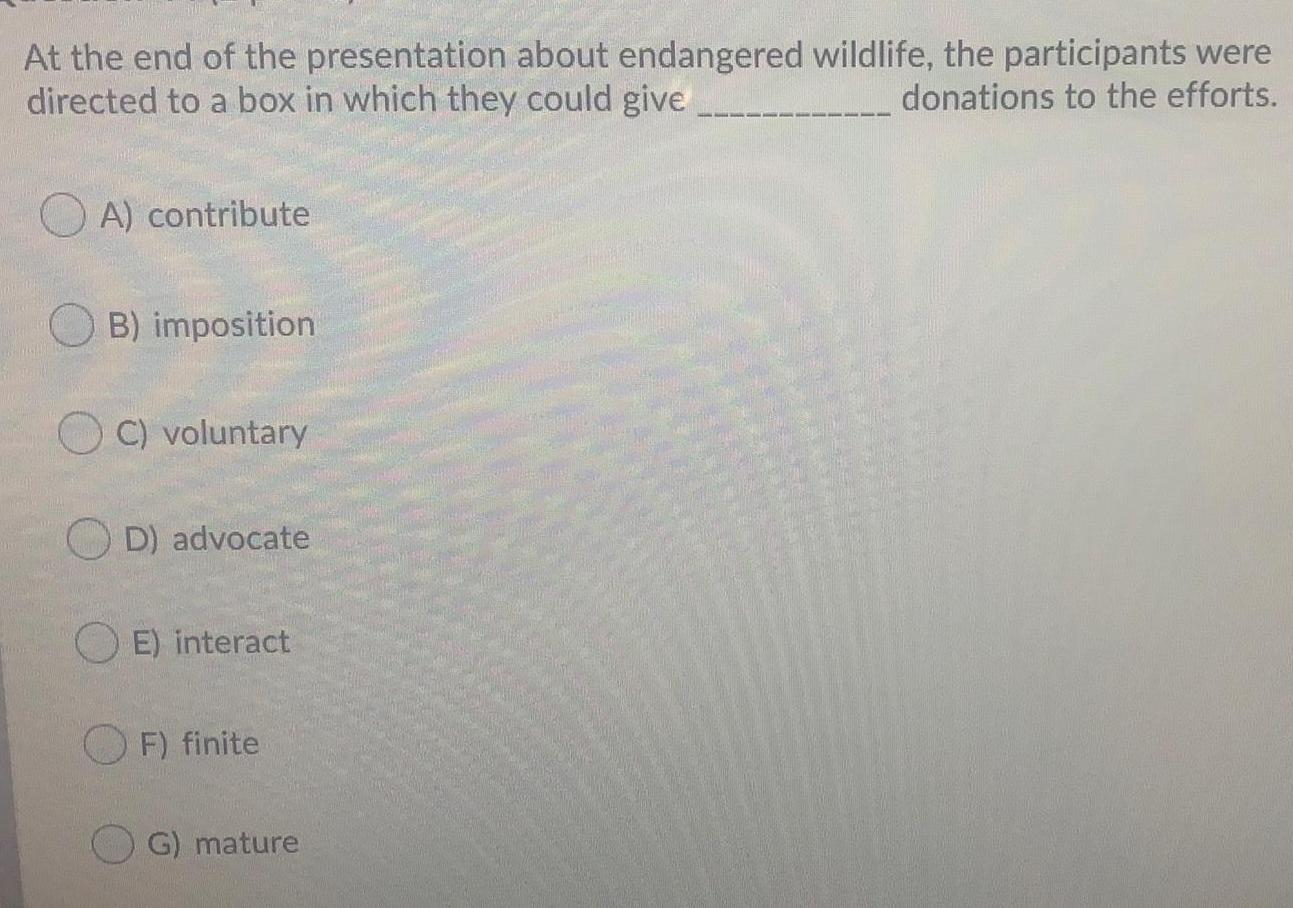Inorganic Chemistry
P Block - Group 17
At the end of the presentation about endangered wildlife, the participants were directed to a box in which they could give donations to the efforts. A) contribute B) imposition C) voluntary D) advocate E) interact F) finite G) mature